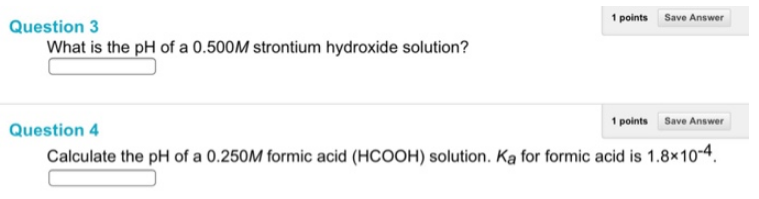# Problem: Question 3What is the pH of a 0.500M strontium hydroxide solution?Question 4Calculate the pH of a 0.250M formic acid (HCOOH) solution. Ka for formic acid is 1.8 x 10-4.

###### FREE Expert Solution
83% (358 ratings)###### Problem Details

Question 3

What is the pH of a 0.500M strontium hydroxide solution?

Question 4

Calculate the pH of a 0.250M formic acid (HCOOH) solution. Ka for formic acid is 1.8 x 10-4.Frequently Asked Questions

What scientific concept do you need to know in order to solve this problem?

Our tutors have indicated that to solve this problem you will need to apply the Weak Acids concept. If you need more Weak Acids practice, you can also practice Weak Acids practice problems.